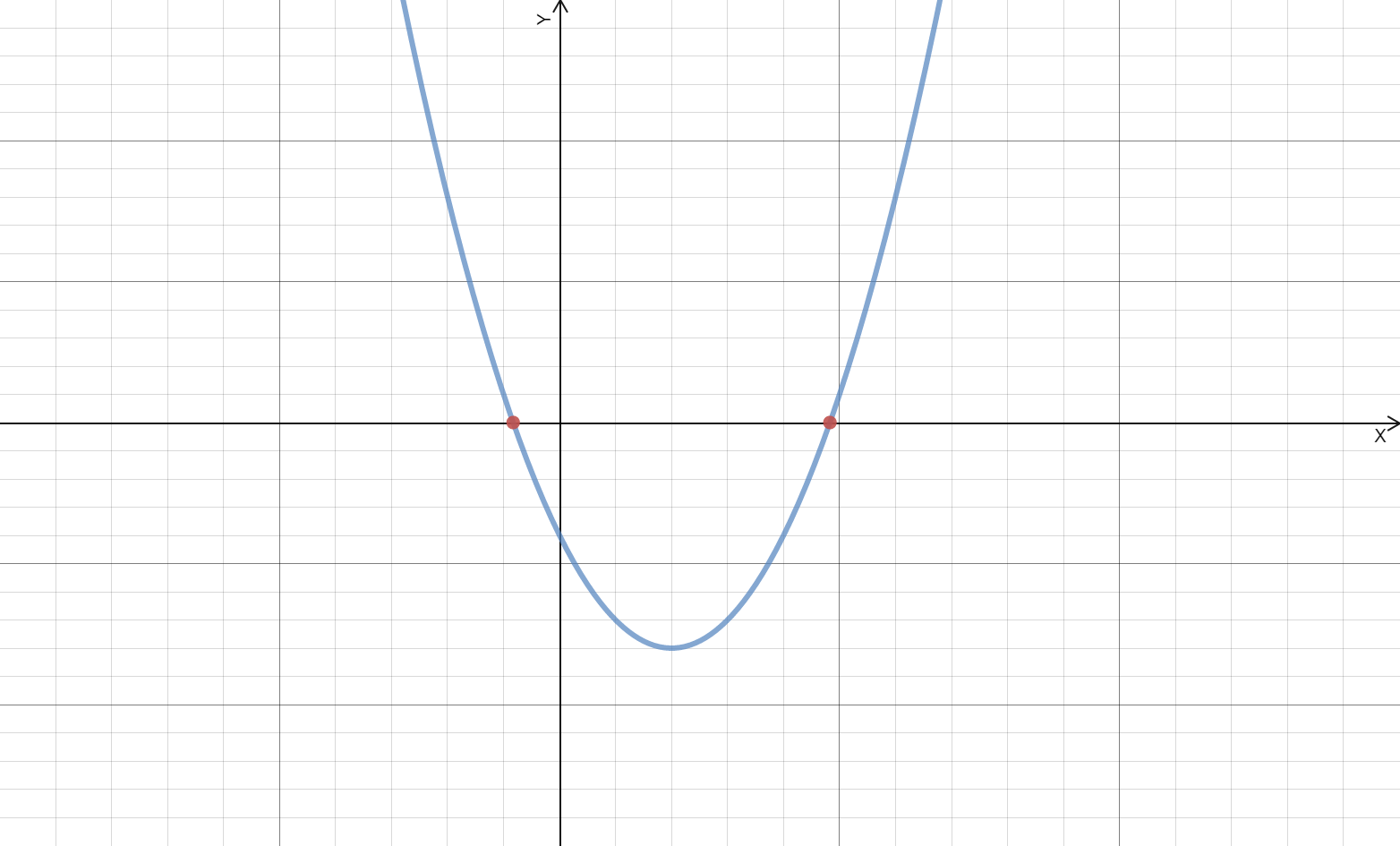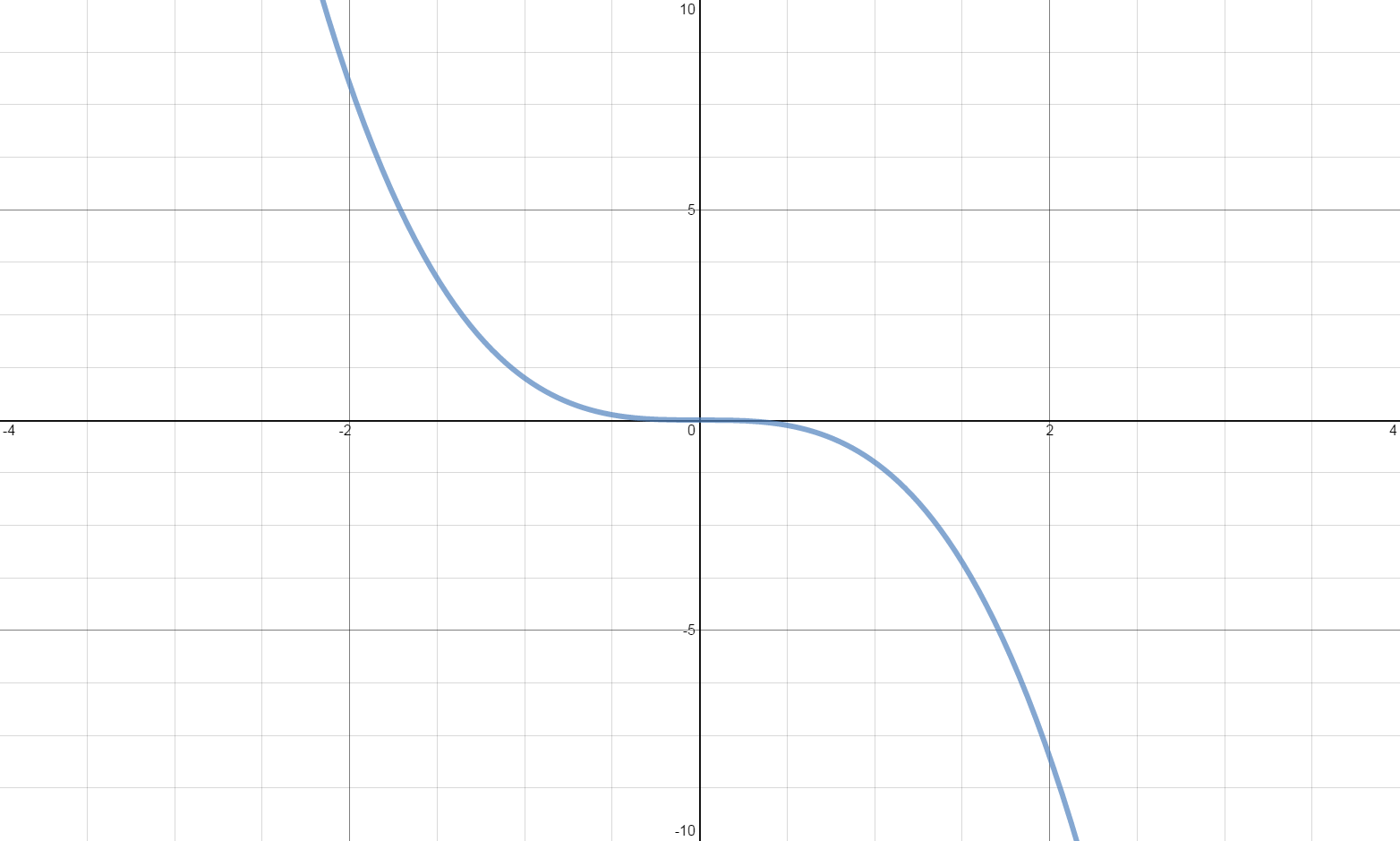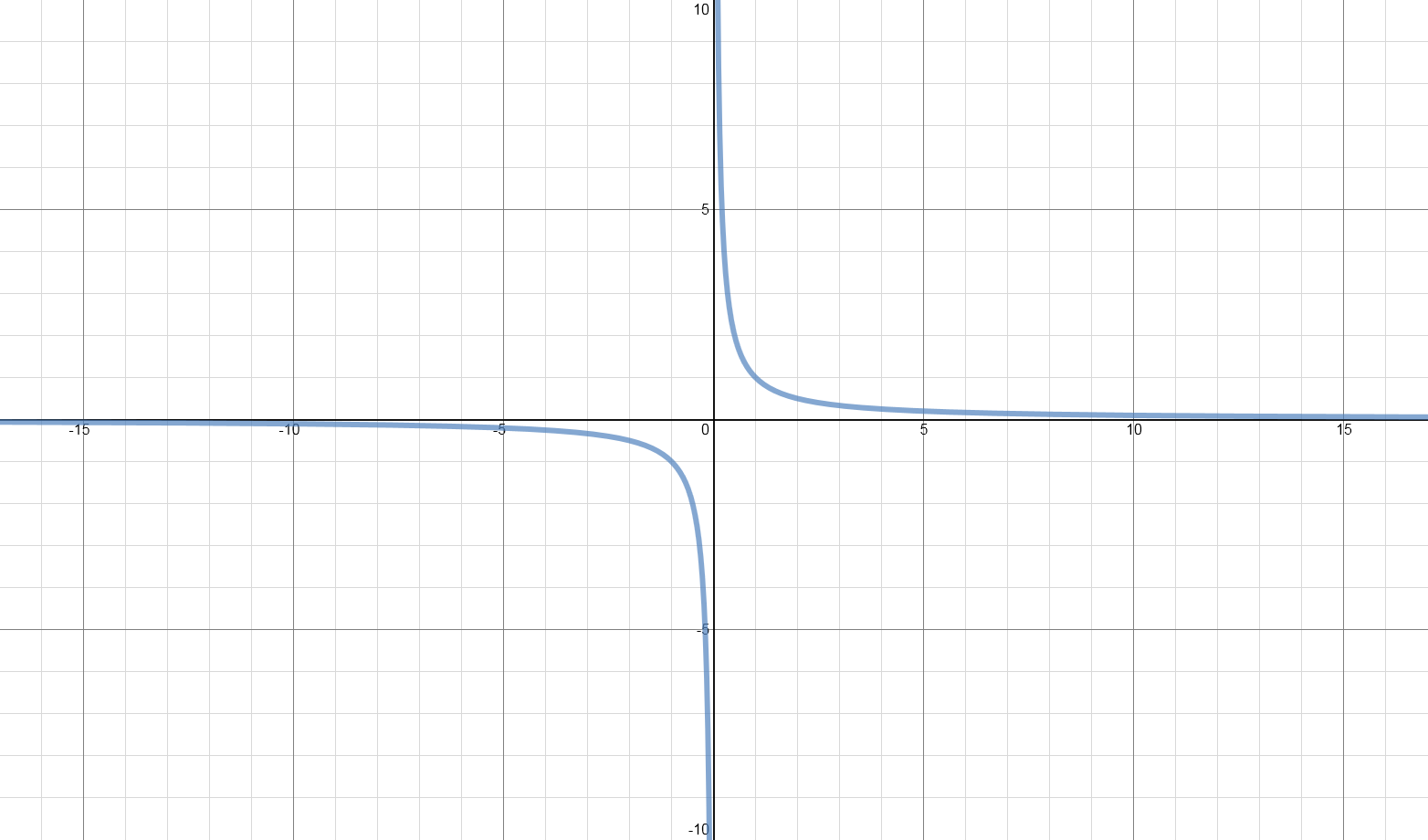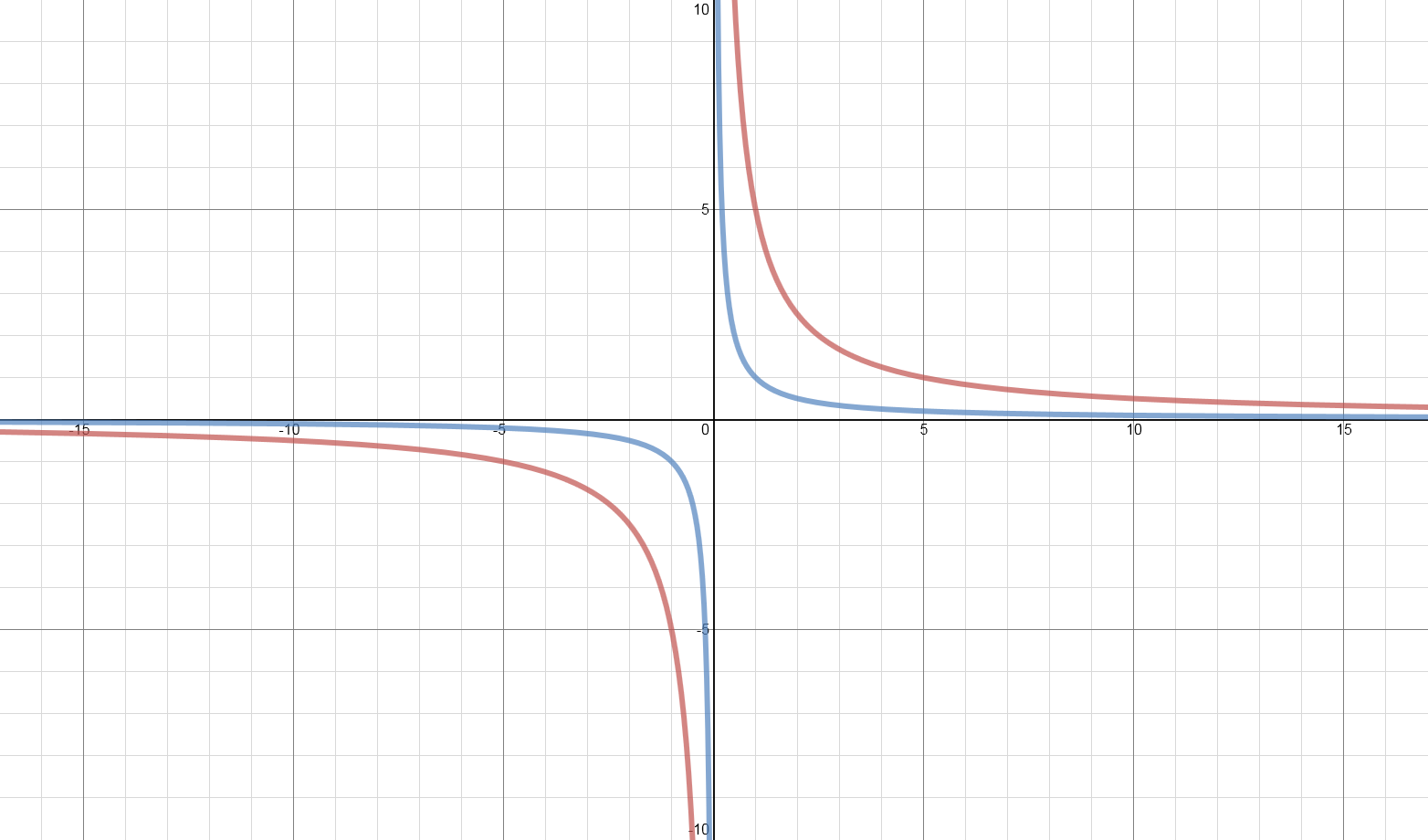Learning Goal

Syllabus Requirement

Students need to understand the graphs of functions including:

•    Sketching curves defined by simple equations.
•    Geometrical interpretation of algebraic solution of equations.
•    Use of intersection points of graphs of functions to solve equations.
•    Functions to include simple cubic functions and the reciprocal fucntion ??y = kx?? with ??x \neq 0??.
•    Knowledge of the term asymptote is expected.

Study Notes

In addition to sketching quadratic curves (discussed in an earlier part of the notes), you should be able to sketch simple linear functions, cubic functions, the reciprocal function and be able to make a sketch of some other function using the same techniques.

Sketching Linear Functions

To graph a linear function you just need to find two points on the line and then draw a line that passes through those two points. The two most sensible points to choose are where the line crosses each axis (if it does cross both) - these can be found by setting ??x=0?? and ??y=0??. However, any two points on the line will do, so you can pick any two values for ??x?? or ??y??.

Every quadratic function will have a graph that is a parabola, or in simpler words, a "u-shape".
Considering the quadratic function in the form ??ax^{2} + bx + c = 0?? can help us sketch the graph.
The figure below shows the general shape of a quadratic function where ??a?? is positive:The graph of ??y=x^{2}??.

When the ??a?? term is negative, the shape of the parabola is flipped to be upside-down as shown in the figure below:The graph of ??y=-x^{2}??.

Next by checking the discriminant we can determine the number of real roots and hence if the curve crosses the ??x?? axis.
Calculate ??b^{2}-4ac?? if:
1. Positive ??\implies?? the function has two real solutions and will therefore intersect the ??x?? axis twice.
2.3. Zero ??\implies?? then the function has a single real solution and will touch the ??x?? axis once. This will also be the turning point of the function.
4.5. Negative ??\implies?? then the function only has complex solutions and will not cross the ??x?? axis.
6.Sketching Cubic Functions

There are several steps to graphing a cubic function:
1. Start by noting whether the coefficient of ??x^{3}?? is positive or negative. If the coefficient is positive, then the graph of ??x^{3}?? will increase rapidly as ??x?? gets very positive and will decrease rapidly ax ??x?? gets very negative. If the coefficient is negative, then the graph of ??x^{3}?? will decrease rapidly as ??x?? gets very positive and will increase rapidly as ??x?? gets very negative. In practice this means you only have to worry about sketchning the middle part of the curve, the outer parts are defined by the coefficient of ??x^{3}??.
2. Find the ??y?? intercept, by setting ??x=0?? in the cubic.
3. Find the roots of the equation to find the ??x?? intercepts.
4. Using all the points you have found, and the general shape of cubic graphs, shown below, sketch the chart.
5. Note that you may be required to, but won't necessarily be, label the turning points of the cubic graph, which can be found by differentiating the cubic and setting the resultant expression to 0.

There are up to three different roots for a cubic function - though in cases one or more of these may coincide. The general shape of cubic charts are shown below for positive and negative coefficients of ??x^{3}??, for the various numbers of different roots.The graph of ??y = x^{3}??. A positive coefficient of ??x^{3}?? with 1 unique root.The graph of ??y = -x^{3}??. A negative coefficient of ??x^{3}?? with 1 unique root.The graph of ??y = x^{3} + 3x^{2}??. A positive coefficient of ??x^{3}?? with 2 unique roots.The graph of ??y = -x^{3} + 3x^{2}??. A negative coefficient of ??x^{3}?? with 2 unique roots.The graph of ??y = x^{3} - x^{2} - x??. A positive coefficient of ??x^{3}?? with 3 unique roots.The graph of ??y = -x^{3} + x^{2} + x??. A negative coefficient of ??x^{3}?? with 3 unique roots.

Sketching Reciprocal Functions

Functions of the form ??\frac{k}{x}?? where ??k?? is a constant and ??x \neq 0?? are called reciprocals.
Reciprocal graphs all have the same shape, which has the following characteristics:

1. The graph gets closer to the ??x??-axis as the value of ??x?? increases (both positively and negatively), but it never reaches the ??x?? axis, this is called the horizontal asymptote.
2. The graph also never reaches the ??y??-axis but approaches it as ??x?? approaches ??0??, this called the vertical asymptote.
3. Graphs of this type of function are symmetrical about two lines: ??y=x?? and ??y=-x??.
Below is the graph for the reciprocal function ??\frac{k}{x}??, where ??\color{Blue}{k=1}??The graphs of ??\color{Blue}{y = \frac{1}{x}}??.

4. If the value of ??k<0?? the graph is mirrored about the ??y?? axis.
Below is the graph for the reciprocal function ??\frac{k}{x}??, where ??\color{Green}{k=-1}??.The graphs of ??\color{Green}{y = -\frac{1}{x}}??.

5. The value of ??k?? does not change the asymptotes, but does change how quickly the graph reaches them.
Below are the graphs of two reciprocal functions of the form ??\frac{k}{x}??, for ??\color{Blue}{k=1}?? and ??\color{Red}{k=5}??:The graphs of ??\color{Blue}{y = \frac{1}{x}}?? and ??\color{Red}{y = \frac{5}{x}}??.

Intersection Points Of Graphs To Solve Equations

An alternative approach to solving simultaneous equations is to solve them graphically. If you plot the graphs of two functions, then the point, or points, at which they cross provide a solution to both equations. The ??x?? and ??y?? coordinates can simply be read off the graph for the solution.

Worked Examples

We'll now run through an example of each of the above types of problem:

Sketching Linear Graphs

Example 1

Question

Sketch the graph of ??y = 2x + 8??.

Sketching Cubic Graphs

Example 2

Question

Sketch the graph of ??y = x(x+3)(x+2)??.

Intersection Points Of Graphs To Solve Equations

Example 3

Question

Graphically, solve the two simultaneous equations: $$y = -x + 5$$ $$y = 3x - 7$$

Exam Questions

The table below contains every exam question that has been asked on this topic, this includes normal papers from both January and June sittings, International papers and Specimen papers.

To see an exam question and solution simply click on the load question icon (), the question will appear below the table and the solution can be shown by clicking the "Show Solution" button that also appears.

The full exam paper and mark schemes are also available for download by clicking on the download icons in each row of the table ().

Question
Exam Board Subject Paper Year Month Module Question
No.
Parts Total
Marks
Exam
Paper
Mark
Scheme
Edexcel Maths Standard 2006 January Core 1 10 (b) 3
Edexcel Maths Standard 2006 June Core 1 3 (a) 3
Edexcel Maths Standard 2006 June Core 1 9 (c) 3
Edexcel Maths Standard 2007 January Core 1 10 (a) 6
Edexcel Maths Standard 2007 June Core 1 9 (c) 3
Edexcel Maths Standard 2008 January Core 1 10 (a) 4
Edexcel Maths Standard 2008 June Core 1 6 (a) 3
Edexcel Maths Standard 2009 January Core 1 8 (a), (b) 6
Edexcel Maths Standard 2009 June Core 1 10 (b) 4
Edexcel Maths Standard 2010 January Core 1 9 (b) 3
Edexcel Maths Standard 2010 January Core 1 10 (c) 3
Edexcel Maths Standard 2010 June Core 1 4 (b) 2
Edexcel Maths Standard 2010 June Core 1 10 (a) 5
Edexcel Maths Standard 2011 January Core 1 10 n/a 8
Edexcel Maths Standard 2011 June Core 1 10 (a) 4
Edexcel Maths Standard 2012 January Core 1 5 (b) 4
Edexcel Maths Standard 2012 January Core 1 8 (b) 3
Edexcel Maths Standard 2012 June Core 1 8 (c) 3
Edexcel Maths Standard 2013 January Core 1 10 (b) 4
Edexcel Maths Standard 2013 June Core 1 11 (a), (b) 3
Edexcel Maths International 2013 June Core 1 9 (a) 5

Exam Tips

1. When attempting to sketch a graph of a function take the following general approach:
Recognise what type of function the question is referring to, either linear, quadratic, cubic or reciprocal
Learn and recall the general shape of the function you are attempting to sketch
Attempt to find any locations at which the curve crosses either axis
For a more accurate sketch calculate the turning points however this is rarely specified in the question
You should clearly state the intercepts in your solutions and check you have provided all of the points in the question

2. The majority of exam questions will ask for the ??x?? axis intercepts therefore practice factorising equations so you can quickly find the roots

3. When sketching a quadratic checking the discriminant (??b^{2}-4ac??) will help you determine if and how many times the curve crosses the ??x?? axis.
Positive ??\implies?? the function has two real solutions and will therefore intersect the ??x?? axis twice.
Zero ??\implies?? then the function has a single real solution and will touch the ??x?? axis once. This will also be the turning point of the function.
Negative ??\implies?? then the function only has complex solutions and will not cross the ??x?? axis.